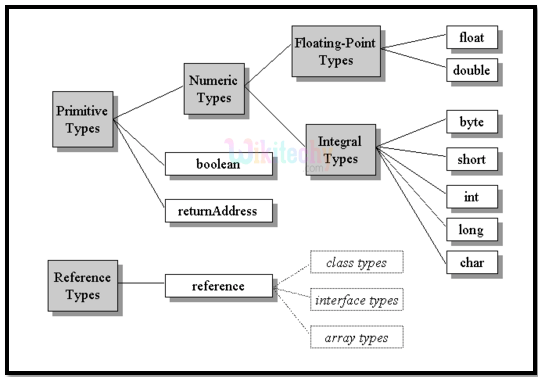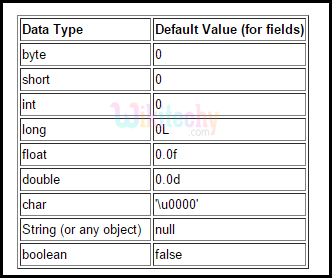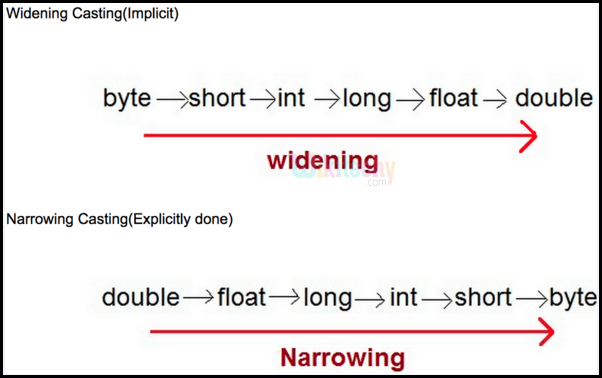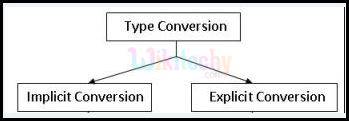# Java - Data types part1

## Java - Data types part1

• Data Type could be a special keyword accustomed to assign adequate memory space for the data
• In other words, it is a form of data representation in main memory (RAM).
• Data Types are of two forms:
1. Primitive.
2. Non –Primitive or Reference Types.## Primitive Data Types :

Primitive data types are predefined by the Java language and are identified by a keyword. Following are the Primitive Data Types:
• Byte
• Short
• Int
• Long
• Float
• Double
• Boolean
• Charshort :

• 16-bit signed integer.
• Minimum value that a short can hold: -32,768 (-2^15)
• Maximum value that a short can hold: 32,767 (2^15 -1)
• Default value for a short: 0.
• Two times smaller than an integer and hence saves memory
• Sample Values: short s = 10000, short r = -10000
###### int :
• 32-bit signed integer.
• Minimum value that an int can hold:  - 2,147,483,648 (-2^31)
• Maximum value that an int can hold:  2,147,483,647 (2^31 -1)
• Default value for an int: 0.
• Default data type preferred for integer values.
• Sample values: int a = 100000, int b = -200000
###### long :
• 64-bit signed integer.
• Minimum value that a long can hold: -9,223,372,036,854,775,808 (-2^63)
• Maximum value that a long can hold:   9,223,372,036,854,775,807 (2^63 -1)
• Default value for a long: 0L.
• Preferred type next to integer data type.
• Example: long a = 100000L, long b = -100000L.
###### float :
• A single-precision 32-bit IEEE 754 floating point.
• Basic type to hold decimal or fractional value as it saves memory.
• Default value for a float: 0.0f.
• Sample Value: float ft = 12.5f
###### double :
• Double-precision 64-bit IEEE 754 floating point.
• Preferred data type for storing decimal values.
• Default value for a double: 0.0d.
• Sample Value: doubledbl = 125.8
###### boolean :
• Symbolize one bit of data.
• Possible values: true or false.
• Default value for a Boolean: false.
• Sample: boolean isStudent = true
###### char :
• A single 16-bit Unicode character.
• Minimum value for a char: '\u0000' (or 0).
• Maximum value for a char: '\uffff'
• Stores a single character.
• Sample Value: char chrVal ='Y'
###### Reference Data Type :
• Reference types are created with defined class constructors for accessing objects.
• They are said to be based on a class.
• Stores the address of its variable.
• Also termed as instantiable class, arrays, String, Scanner, Random, Die, int[], String[], etc.

## Type Casting :

• Allocating the value of variable with a type to another variable of varying type.• Up casting - casting a variable to a superior data type, which never costs loss of data. Termed as widening data type.
• Down casting - casting a variable to a lesser data type, which might cause loss of data. Termed as narrowing data type.
Examples :
Up Casting :
int a=9, b=4;
double divVal = (double) a/b;
Down Casting :
int a = 9;
short b = (short) a;

## Up Casting Types :

`byte -> short, int, long, float, doubleshort -> int, long, float, doublechar -> int, long, float, doubleint -> long, float, doublelong -> float, doublefloat -> double `

## Down Casting Types :

```short -> byte, char
char -> byte, short
int -> byte, short, char
long -> byte, short, char, int
float -> byte, short, char, int, long
double -> byte, short, char, int, long, float
```

Type Conversion:
• Three forms, automatic, explicit and Boolean.
• Explicit - casting done by the programmer explicitly for his own purpose.
• Implicit - casting done by the compiler implicitly to complete an operation between two operands of different data types. Up casting is used here for conversion.• Boolean– cannot be cast to any other data type. Excluding Boolean, all other data types can be cast either implicitly or explicitly.

## Example:

Implicit Casting :
int a=9, b=4;
long c = a * b;
Explicit Casting :
short a = 9; char b= ’B’;
short c = (short) (a + b);

Bug Bounty
Webinar